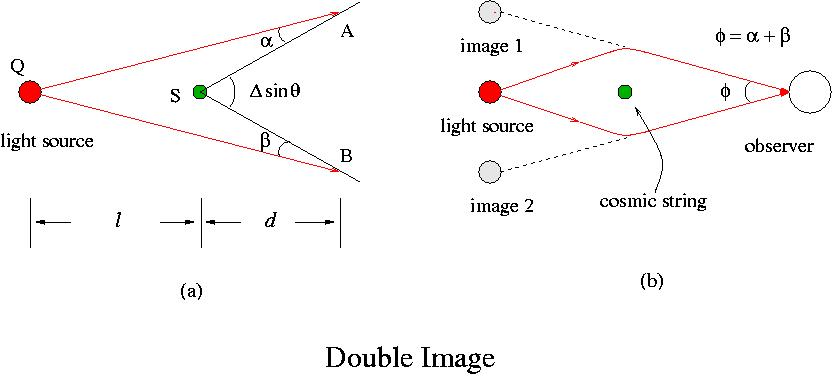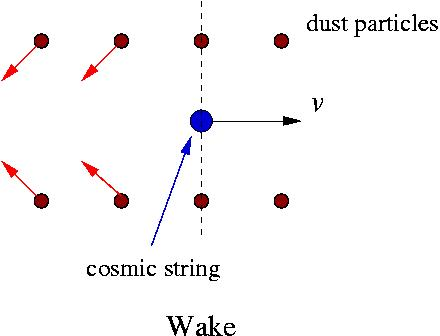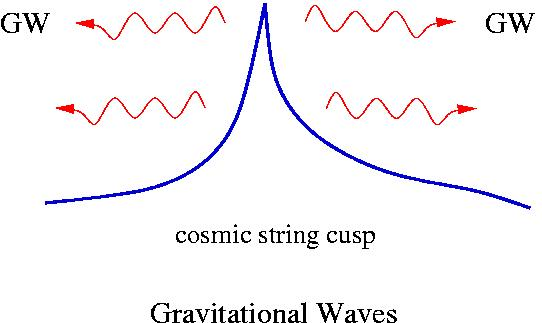# String Dynamics

A cosmic string has very interesting physical effects. All these effects are originated from its unique way to deform the space-time around it.  A model of an infinitely long, straight cosmic string is one of the exactly solvable systems in General Relativity. Since it is made of vacuum energy rather than ordinary matter, it has a different solution of the Einstein equations, a conic space-time!Figure 4. Conic space-time formed around a straight string, One complete turn around the string makes up less than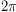by the deficit angle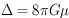.

The metric around a straight string is given by (in cylindrical coordinates)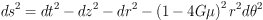where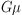is the cosmic string parameter (G=Newton constant,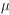= string energy density). It is a flat space-time metric except for the deficit angle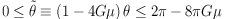.
Motion of a cosmic string can be described by Nambu-Goto action,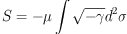and it satisfies wave equation. Cosmic strings are expected to have huge tension, tension ~ mass density in natural unit so that their motions are relativistic.
Possible Physical Effects:
(1) Double Images
(2) Kaiser-Stebbins Effect
(3) Wakes